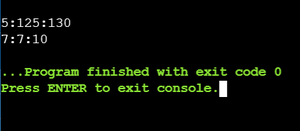GFG App
Open AppBrowser
Continue

# C++ program to convert/normalize the given time into standard form

Given three variables that represent the hours, minutes, and the second, the task is to write a C++ program to normalize/convert the given time into standard form i.e., HR: MIN: SEC format.

Examples:

Input: HR = 5, MIN = 125, SEC = 130
Output: 7:7:10

Approach: To normalize the given time, below are the functions/steps using the class:

• 3 variables are defined to store the value of hours, minutes, and second respectively.
```int HR, MIN, SEC;
where HR represents hours,
MIN represents minutes and
SEC represents seconds```
• setTime() function to set values of HR, MIN and SEC:
```void setTime(int x, int y, int z)
{
x = HR;
y = MIN;
z = SEC;
}```
• showTime() to display the time:
```void showTime()
{
cout << HR << ":" << MIN << ":" << SEC;
}```
• normalize() function to convert the resultant time into standard form. Normalizing the time by converting it according to the standard time format as:
```void normalize()
{
MIN = MIN + SEC/60;
SEC = SEC%60;
HR  = HR + MIN/60;
MIN = MIN%60;
}```

Illustration: For Example Time: 5: 125: 130

• To normalize the time:
• First, divide the SEC by 60 and to find the minutes and add it into MIN,
• so, 125+(130/60) =125+2 = 127.
• Then take the modulo of SEC to find the seconds 130 % 60 = 10.
• Now, divide MIN by 60 find the hours, and add it into HR, 5+(127/60) = 5+2= 7.
• Lastly take modulus of MIN to find the minutes, 127 % 60 = 7, So, HR= 7, MIN= 7, SEC= 10.
• Therefore, the result is 7: 7: 10

Below is the implementation of the above approach:

## C++

 `// C++ program to normalize the given` `// time into standard form` `#include ` `#include ` `using` `namespace` `std;`   `// Time class` `class` `Time {` `private``:` `    ``// Instance variables` `    ``int` `HR, MIN, SEC;`   `public``:` `    ``void` `setTime(``int``, ``int``, ``int``);` `    ``void` `showTime();` `    ``void` `normalize();` `};`   `// Function that sets the given time` `void` `Time::setTime(``int` `h, ``int` `m, ``int` `s)` `{` `    ``HR = h;` `    ``MIN = m;` `    ``SEC = s;` `}`   `// Function to show the given time` `void` `Time::showTime()` `{` `    ``cout << endl` `         ``<< HR << ``":"` `         ``<< MIN << ``":"` `<< SEC;` `}`   `// Function to normalize the given` `// time into standard form` `void` `Time::normalize()` `{` `    ``// Convert the time into the` `    ``// specific format` `    ``MIN = MIN + SEC / 60;` `    ``SEC = SEC % 60;` `    ``HR = HR + MIN / 60;` `    ``MIN = MIN % 60;` `}`   `// Driver Code` `int` `main()` `{` `    ``// Object of class Time` `    ``Time t1;`   `    ``t1.setTime(5, 125, 130);` `    ``t1.showTime();`   `    ``// Normalize the time` `    ``t1.normalize();` `    ``t1.showTime();`   `    ``return` `0;` `}`

Output:My Personal Notes arrow_drop_up